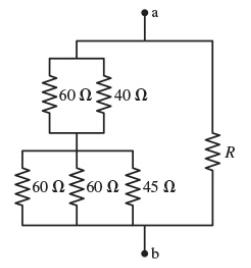# Problem: Suppose that R = 70 Ω.What is the equivalent resistance between the points a and b in Figure 1? Express your answer with the appropriate units.Rab = ____

###### FREE Expert Solution

Equivalent resistance for resistors in series:

$\overline{){{\mathbf{R}}}_{\mathbf{e}\mathbf{q}}{\mathbf{=}}{{\mathbf{R}}}_{{\mathbf{1}}}{\mathbf{+}}{{\mathbf{R}}}_{{\mathbf{2}}}{\mathbf{+}}{\mathbf{.}}{\mathbf{.}}{\mathbf{.}}{\mathbf{+}}{{\mathbf{R}}}_{{\mathbf{n}}}}$

Equivalent resistor for resistors in parallel:

$\overline{)\frac{\mathbf{1}}{{\mathbf{R}}_{\mathbf{e}\mathbf{q}}}{\mathbf{=}}\frac{\mathbf{1}}{{\mathbf{R}}_{\mathbf{1}}}{\mathbf{+}}\frac{\mathbf{1}}{{\mathbf{R}}_{\mathbf{2}}}{\mathbf{+}}{\mathbf{.}}{\mathbf{.}}{\mathbf{.}}{\mathbf{+}}\frac{\mathbf{1}}{{\mathbf{R}}_{\mathbf{n}}}}$

60Ω and 40Ω are in parallel.

1/Req1 = 1/60 + 1/40 = 1/24

Req = 24Ω

60Ω, 60Ω, and 45Ω resistors are in parallel:

1/Req2 = 1/60 + 1/60 + 1/45 = 1/18###### Problem Details

Suppose that R = 70 Ω.What is the equivalent resistance between the points a and b in Figure 1? Express your answer with the appropriate units.

Rab = ____